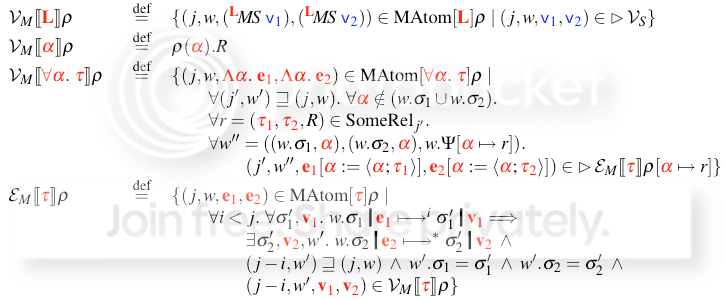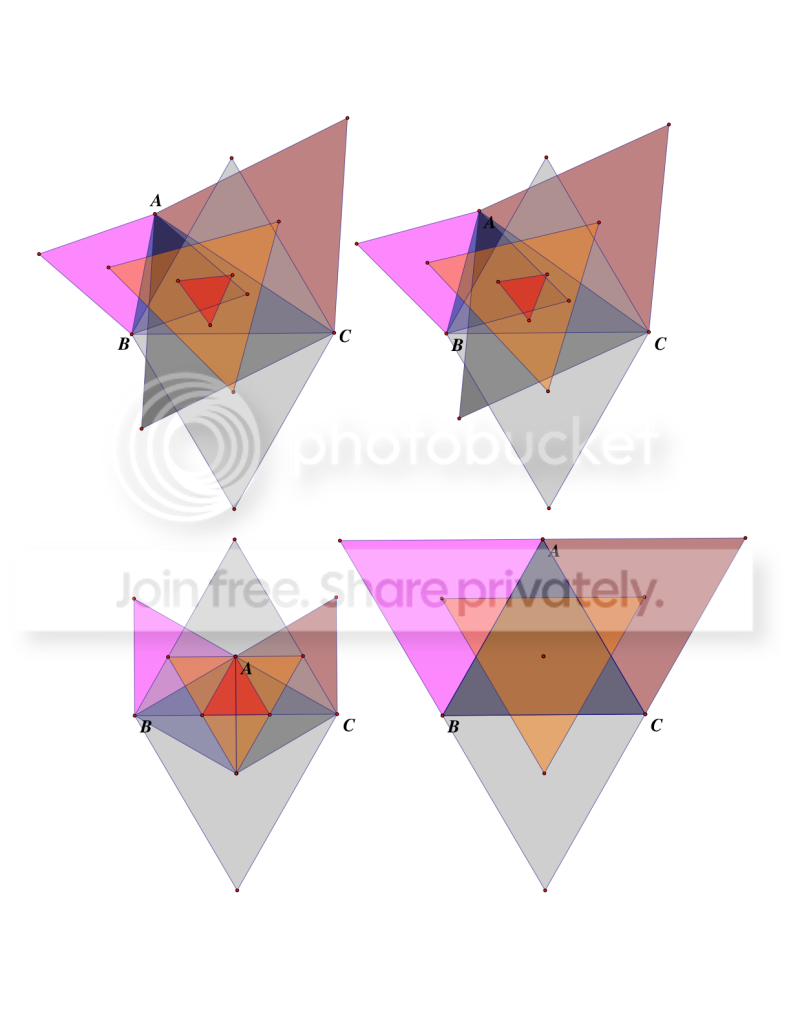# Napoleon's theorem

Most of the math that I deal with for my job looks like this:So, every once in a while, it's refreshing to deal with some math that looks like this:These diagrams illustrate Napoleon's theorem. In the top left diagram, the triangle ABC has no special properties to speak of, but if you construct equilateral triangles pointing outwards from its three sides, then the centers1 of those three triangles form another equilateral triangle. (It's the light orange one in the diagram.) If you construct equilateral triangles pointing inwards from the sides of ABC, and then connect the centers of those, you get another equilateral triangle, centered on the first. (It's the dark orange one.) These are called the Napoleon triangles, and the difference in the areas of the two Napoleon triangles equals the area of ABC!

If ABC is equilateral, then the inner, dark orange Napoleon triangle shrinks to a point, as in the bottom right diagram. The bottom left shows what happens if ABC is isoceles, and the top right shows what happens when I try to make the sides of the two Napoleon triangles parallel. I'm pretty sure that there are infinite points I could move the A vertex to that would cause that to be true, and I think that they might describe a circle.

This has been your daily triangle geometry update. Now, back to bending LaTeX to my will.

1. There are a lot of ways to define the "center" of a triangle. Conveniently, if it's an equilateral triangle, the definitions all agree.
Tags:
• Post a new comment

#### Error

Anonymous comments are disabled in this journal

default userpic